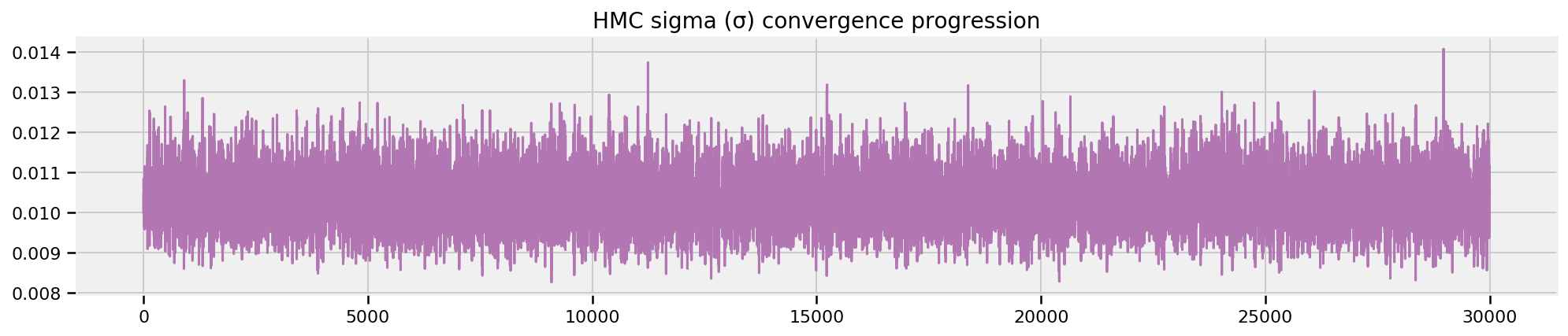# Probabilistic Programming and Bayesian Methods for Hackers Chapter 5¶

Original content (this Jupyter notebook) created by Cam Davidson-Pilon (@Cmrn_DP) and Tim Salimans (@TimSalimans)

Ported to Tensorflow Probability by Matthew McAteer (@MatthewMcAteer0), with help from Bryan Seybold, Mike Shwe (@mikeshwe), Josh Dillon, and the rest of the TFP team at Google ([email protected]).

Welcome to Bayesian Methods for Hackers. The full Github repository is available at github/Probabilistic-Programming-and-Bayesian-Methods-for-Hackers. The other chapters can be found on the project's homepage. We hope you enjoy the book, and we encourage any contributions!

### Table of Contents¶

• Dependencies & Prerequisites
• Would you rather lose and arm or a leg?
• Loss Functions
• Loss functions in the real world
• Example: Optimizing for the Showcase on The Price is Right
• Minimizing our losses
• Shortcuts
• Machine Learning via Bayesian Methods
• Example: Financial prediction
• Example: Kaggle contest on Observing Dark World
• Setup
• Defining our galaxy-plotting function
• Examining Our Data
• Priors
• Training & Tensorflow implemenation
• Constructing a probabilistic model for the data (observed ellipcities o the galaxies) given the positions of the dark matter halos
• Using Bayes' rule to get the posterior distribution of the halo positions, i.e. to use the data to guess wherre the dark matter halos might be
• References

## Loss Functions¶

### Dependencies & Prerequisites¶

Tensorflow Probability is part of the colab default runtime, so you don't need to install Tensorflow or Tensorflow Probability if you're running this in the colab.
If you're running this notebook in Jupyter on your own machine (and you have already installed Tensorflow), you can use the following
• For the most recent nightly installation: pip3 install -q tfp-nightly
• For the most recent stable TFP release: pip3 install -q --upgrade tensorflow-probability
• For the most recent stable GPU-connected version of TFP: pip3 install -q --upgrade tensorflow-probability-gpu
• For the most recent nightly GPU-connected version of TFP: pip3 install -q tfp-nightly-gpu
Again, if you are running this in a Colab, Tensorflow and TFP are already installed
In :
#@title Imports and Global Variables  { display-mode: "form" }
"""
The book uses a custom matplotlibrc file, which provides the unique styles for
matplotlib plots. If executing this book, and you wish to use the book's
styling, provided are two options:
1. Overwrite your own matplotlibrc file with the rc-file provided in the
book's styles/ dir. See http://matplotlib.org/users/customizing.html
2. Also in the styles is  bmh_matplotlibrc.json file. This can be used to
update the styles in only this notebook. Try running the following code:

import json
s = json.load(open("../styles/bmh_matplotlibrc.json"))
matplotlib.rcParams.update(s)
"""
!pip3 install -q wget
from __future__ import absolute_import, division, print_function

#@markdown This sets the warning status (default is ignore, since this notebook runs correctly)
warning_status = "ignore" #@param ["ignore", "always", "module", "once", "default", "error"]
import warnings
warnings.filterwarnings(warning_status)
with warnings.catch_warnings():
warnings.filterwarnings(warning_status, category=DeprecationWarning)
warnings.filterwarnings(warning_status, category=UserWarning)

import numpy as np
import os
#@markdown This sets the styles of the plotting (default is styled like plots from [FiveThirtyeight.com](https://fivethirtyeight.com/))
matplotlib_style = 'fivethirtyeight' #@param ['fivethirtyeight', 'bmh', 'ggplot', 'seaborn', 'default', 'Solarize_Light2', 'classic', 'dark_background', 'seaborn-colorblind', 'seaborn-notebook']
import matplotlib.pyplot as plt; plt.style.use(matplotlib_style)
import matplotlib.axes as axes;
from matplotlib.patches import Ellipse
from mpl_toolkits.mplot3d import Axes3D
%matplotlib inline
import seaborn as sns; sns.set_context('notebook')
from scipy.optimize import fmin
from IPython.core.pylabtools import figsize
#@markdown This sets the resolution of the plot outputs (retina is the highest resolution)
notebook_screen_res = 'retina' #@param ['retina', 'png', 'jpeg', 'svg', 'pdf']
%config InlineBackend.figure_format = notebook_screen_res

import tensorflow as tf
tfe = tf.contrib.eager

# Eager Execution
#@markdown Check the box below if you want to use [Eager Execution](https://www.tensorflow.org/guide/eager)
#@markdown Eager execution provides An intuitive interface, Easier debugging, and a control flow comparable to Numpy. You can read more about it on the [Google AI Blog](https://ai.googleblog.com/2017/10/eager-execution-imperative-define-by.html)
use_tf_eager = False #@param {type:"boolean"}

# Use try/except so we can easily re-execute the whole notebook.
if use_tf_eager:
try:
tf.enable_eager_execution()
except:
pass

import tensorflow_probability as tfp
tfd = tfp.distributions
tfb = tfp.bijectors

def evaluate(tensors):
"""Evaluates Tensor or EagerTensor to Numpy ndarrays.
Args:
tensors: Object of Tensor or EagerTensors; can be list, tuple,
namedtuple or combinations thereof.

Returns:
ndarrays: Object with same structure as tensors except with Tensor or
EagerTensors replaced by Numpy ndarrays.
"""
if tf.executing_eagerly():
return tf.contrib.framework.nest.pack_sequence_as(
tensors,
[t.numpy() if tf.contrib.framework.is_tensor(t) else t
for t in tf.contrib.framework.nest.flatten(tensors)])
return sess.run(tensors)

class _TFColor(object):
"""Enum of colors used in TF docs."""
red = '#F15854'
blue = '#5DA5DA'
orange = '#FAA43A'
green = '#60BD68'
pink = '#F17CB0'
brown = '#B2912F'
purple = '#B276B2'
yellow = '#DECF3F'
gray = '#4D4D4D'
def __getitem__(self, i):
return [
self.red,
self.orange,
self.green,
self.blue,
self.pink,
self.brown,
self.purple,
self.yellow,
self.gray,
][i % 9]
TFColor = _TFColor()

def session_options(enable_gpu_ram_resizing=True, enable_xla=True):
"""
Allowing the notebook to make use of GPUs if they're available.

XLA (Accelerated Linear Algebra) is a domain-specific compiler for linear
algebra that optimizes TensorFlow computations.
"""
config = tf.ConfigProto()
config.log_device_placement = True
if enable_gpu_ram_resizing:
# allow_growth=True makes it possible to connect multiple colabs to your
# GPU. Otherwise the colab malloc's all GPU ram.
config.gpu_options.allow_growth = True
if enable_xla:
# Enable on XLA. https://www.tensorflow.org/performance/xla/.
config.graph_options.optimizer_options.global_jit_level = (
tf.OptimizerOptions.ON_1)
return config

def reset_sess(config=None):
"""
Convenience function to create the TF graph & session or reset them.
"""
if config is None:
config = session_options()
global sess
tf.reset_default_graph()
try:
sess.close()
except:
pass
sess = tf.InteractiveSession(config=config)

reset_sess()


The default version of TensorFlow in Colab will soon switch to TensorFlow 2.x.
We recommend you upgrade now or ensure your notebook will continue to use TensorFlow 1.x via the %tensorflow_version 1.x magic: more info.

WARNING:tensorflow:
The TensorFlow contrib module will not be included in TensorFlow 2.0.
For more information, please see:
* https://github.com/tensorflow/community/blob/master/rfcs/20180907-contrib-sunset.md
* https://github.com/tensorflow/addons
* https://github.com/tensorflow/io (for I/O related ops)
If you depend on functionality not listed there, please file an issue.

Device mapping:
/job:localhost/replica:0/task:0/device:XLA_CPU:0 -> device: XLA_CPU device
/job:localhost/replica:0/task:0/device:XLA_GPU:0 -> device: XLA_GPU device
/job:localhost/replica:0/task:0/device:GPU:0 -> device: 0, name: Tesla P100-PCIE-16GB, pci bus id: 0000:00:04.0, compute capability: 6.0



### Would you rather lose an arm or a leg?¶

Statisticians can be a sour bunch. Instead of considering their winnings, they only measure how much they have lost. In fact, they consider their wins as negative losses. But what's interesting is how they measure their losses.

For example, consider the following example:

A meteorologist is predicting the probability of a possible hurricane striking his city. He estimates, with 95% confidence, that the probability of it not striking is between 99% - 100%. He is very happy with his precision and advises the city that a major evacuation is unnecessary. Unfortunately, the hurricane does strike and the city is flooded.

This stylized example shows the flaw in using a pure accuracy metric to measure outcomes. Using a measure that emphasizes estimation accuracy, while an appealing and objective thing to do, misses the point of why you are even performing the statistical inference in the first place: results of inference. The author Nassim Taleb of The Black Swan and Antifragility stresses the importance of the payoffs of decisions, not the accuracy. Taleb distills this quite succinctly: "I would rather be vaguely right than very wrong."

## Loss Functions¶

We introduce what statisticians and decision theorists call loss functions. A loss function is a function of the true parameter, and an estimate of that parameter

$$L( \theta, \hat{\theta} ) = f( \theta, \hat{\theta} )$$

The important point of loss functions is that it measures how bad our current estimate is: the larger the loss, the worse the estimate is according to the loss function. A simple, and very common, example of a loss function is the squared-error loss:

$$L( \theta, \hat{\theta} ) = ( \theta - \hat{\theta} )^2$$

The squared-error loss function is used in estimators like linear regression, UMVUEs and many areas of machine learning. We can also consider an asymmetric squared-error loss function, something like:

$$L( \theta, \hat{\theta} ) = \begin{cases} ( \theta - \hat{\theta} )^2 & \hat{\theta} \lt \theta \\\\ c( \theta - \hat{\theta} )^2 & \hat{\theta} \ge \theta, \;\; 0\lt c \lt 1 \end{cases}$$

which represents that estimating a value larger than the true estimate is preferable to estimating a value below. A situation where this might be useful is in estimating web traffic for the next month, where an over-estimated outlook is preferred so as to avoid an underallocation of server resources.

A negative property about the squared-error loss is that it puts a disproportionate emphasis on large outliers. This is because the loss increases quadratically, and not linearly, as the estimate moves away. That is, the penalty of being three units away is much less than being five units away, but the penalty is not much greater than being one unit away, though in both cases the magnitude of difference is the same:

$$\frac{1^2}{3^2} \lt \frac{3^2}{5^2}, \;\; \text{although} \;\; 3-1 = 5-3$$

This loss function imposes that large errors are very bad. A more robust loss function that increases linearly with the difference is the absolute-loss

$$L( \theta, \hat{\theta} ) = | \theta - \hat{\theta} |$$

Other popular loss functions include:

• $L( \theta, \hat{\theta} ) = \mathbb{1}_{ \hat{\theta} \neq \theta }$ is the zero-one loss often used in machine learning classification algorithms.
• $L( \theta, \hat{\theta} ) = -\theta\log( \hat{\theta} ) - (1- \theta)\log( 1 - \hat{\theta} ), \; \; \theta \in {0,1}, \; \hat{\theta} \in [0,1]$, called the log-loss, also used in machine learning.

Historically, loss functions have been motivated from 1) mathematical convenience, and 2) they are robust to application, i.e., they are objective measures of loss. The first reason has really held back the full breadth of loss functions. With computers being agnostic to mathematical convenience, we are free to design our own loss functions, which we take full advantage of later in this Chapter.

With respect to the second point, the above loss functions are indeed objective, in that they are most often a function of the difference between estimate and true parameter, independent of signage or payoff of choosing that estimate. This last point, its independence of payoff, causes quite pathological results though. Consider our hurricane example above: the statistician equivalently predicted that the probability of the hurricane striking was between 0% to 1%. But if he had ignored being precise and instead focused on outcomes (99% chance of no flood, 1% chance of flood), he might have advised differently.

By shifting our focus from trying to be incredibly precise about parameter estimation to focusing on the outcomes of our parameter estimation, we can customize our estimates to be optimized for our application. This requires us to design new loss functions that reflect our goals and outcomes. Some examples of more interesting loss functions:

• $L( \theta, \hat{\theta} ) = \frac{ | \theta - \hat{\theta} | }{ \theta(1-\theta) }, \; \; \hat{\theta}, \theta \in [0,1]$ emphasizes an estimate closer to 0 or 1 since if the true value $\theta$ is near 0 or 1, the loss will be very large unless $\hat{\theta}$ is similarly close to 0 or 1. This loss function might be used by a political pundit whose job requires him or her to give confident "Yes/No" answers. This loss reflects that if the true parameter is close to 1 (for example, if a political outcome is very likely to occur), he or she would want to strongly agree as to not look like a skeptic.

• $L( \theta, \hat{\theta} ) = 1 - \exp \left( -(\theta - \hat{\theta} )^2 \right)$ is bounded between 0 and 1 and reflects that the user is indifferent to sufficiently-far-away estimates. It is similar to the zero-one loss above, but not quite as penalizing to estimates that are close to the true parameter.

• Complicated non-linear loss functions can programmed:
def loss(true_value, estimate):
if estimate*true_value > 0:
return abs(estimate - true_value)
else:
return abs(estimate)*(estimate - true_value)**2

• Another example is from the book The Signal and The Noise. Weather forecasters have an interesting loss function for their predictions.

People notice one type of mistake — the failure to predict rain — more than other, false alarms. If it rains when it isn't supposed to, they curse the weatherman for ruining their picnic, whereas an unexpectedly sunny day is taken as a serendipitous bonus.

[The Weather Channel's bias] is limited to slightly exaggerating the probability of rain when it is unlikely to occur — saying there is a 20 percent change when they know it is really a 5 or 10 percent chance — covering their butts in the case of an unexpected sprinkle.

As you can see, loss functions can be used for good and evil: with great power, comes great — well you know.

## Loss functions in the real world¶

So far we have been under the unrealistic assumption that we know the true parameter. Of course if we knew the true parameter, bothering to guess an estimate is pointless. Hence a loss function is really only practical when the true parameter is unknown.

In Bayesian inference, we have a mindset that the unknown parameters are really random variables with prior and posterior distributions. Concerning the posterior distribution, a value drawn from it is a possible realization of what the true parameter could be. Given that realization, we can compute a loss associated with an estimate. As we have a whole distribution of what the unknown parameter could be (the posterior), we should be more interested in computing the expected loss given an estimate. This expected loss is a better estimate of the true loss than comparing the given loss from only a single sample from the posterior.

First it will be useful to explain a Bayesian point estimate. The systems and machinery present in the modern world are not built to accept posterior distributions as input. It is also rude to hand someone over a distribution when all they asked for was an estimate. In the course of an individual's day, when faced with uncertainty we still act by distilling our uncertainty down to a single action. Similarly, we need to distill our posterior distribution down to a single value (or vector in the multivariate case). If the value is chosen intelligently, we can avoid the flaw of frequentist methodologies that mask the uncertainty and provide a more informative result.The value chosen, if from a Bayesian posterior, is a Bayesian point estimate.

Suppose $P(\theta | X)$ is the posterior distribution of $\theta$ after observing data $X$, then the following function is understandable as the expected loss of choosing estimate $\hat{\theta}$ to estimate $\theta$:

$$l(\hat{\theta} ) = E_{\theta}\left[ \; L(\theta, \hat{\theta}) \; \right]$$

This is also known as the risk of estimate $\hat{\theta}$. The subscript $\theta$ under the expectation symbol is used to denote that $\theta$ is the unknown (random) variable in the expectation, something that at first can be difficult to consider.

We spent all of last chapter discussing how to approximate expected values. Given $N$ samples $\theta_i,\; i=1,...,N$ from the posterior distribution, and a loss function $L$, we can approximate the expected loss of using estimate $\hat{\theta}$ by the Law of Large Numbers:

$$\frac{1}{N} \sum_{i=1}^N \;L(\theta_i, \hat{\theta} ) \approx E_{\theta}\left[ \; L(\theta, \hat{\theta}) \; \right] = l(\hat{\theta} )$$

Notice that measuring your loss via an expected value uses more information from the distribution than the MAP estimate which, if you recall, will only find the maximum value of the distribution and ignore the shape of the distribution. Ignoring information can over-expose yourself to tail risks, like the unlikely hurricane, and leaves your estimate ignorant of how ignorant you really are about the parameter.

Similarly, compare this with frequentist methods, that traditionally only aim to minimize the error, and do not consider the loss associated with the result of that error. Compound this with the fact that frequentist methods are almost guaranteed to never be absolutely accurate. Bayesian point estimates fix this by planning ahead: your estimate is going to be wrong, you might as well err on the right side of wrong.

## Example: Optimizing for the Showcase on The Price is Right¶

Bless you if you are ever chosen as a contestant on the Price is Right, for here we will show you how to optimize your final price on the Showcase. For those who forget the rules:

1. Two contestants compete in The Showcase.
2. Each contestant is shown a unique suite of prizes.
3. After the viewing, the contestants are asked to bid on the price for their unique suite of prizes.
4. If a bid price is over the actual price, the bid's owner is disqualified from winning.
5. If a bid price is under the true price by less than $250, the winner is awarded both prizes. The difficulty in the game is balancing your uncertainty in the prices, keeping your bid low enough so as to not bid over, and trying to bid close to the price. Suppose we have recorded the Showcases from previous The Price is Right episodes and have prior beliefs about what distribution the true price follows. For simplicity, suppose it follows a Normal: $$\text{True Price} \sim \text{Normal}(\mu_p, \sigma_p )$$ In a later chapter, we will actually use real Price is Right Showcase data to form the historical prior, but this requires some advanced Tensorflow use so we will not use it here. For now, we will assume$\mu_p = 35 000$and$\sigma_p = 7500$. We need a model of how we should be playing the Showcase. For each prize in the prize suite, we have an idea of what it might cost, but this guess could differ significantly from the true price. (Couple this with increased pressure being onstage and you can see why some bids are so wildly off). Let's suppose your beliefs about the prices of prizes also follow Normal distributions: $$\text{Prize}_i \sim \text{Normal}(\mu_i, \sigma_i ),\;\; i=1,2$$ This is really why Bayesian analysis is great: we can specify what we think a fair price is through the$\mu_i$parameter, and express uncertainty of our guess in the$\sigma_i$parameter. We'll assume two prizes per suite for brevity, but this can be extended to any number. The true price of the prize suite is then given by$\text{Prize}_1 + \text{Prize}_2 + \epsilon$, where$\epsilon$is some error term. We are interested in the updated$\text{True Price}given we have observed both prizes and have belief distributions about them. We can perform this using Tensorflow Probability. Lets make some values concrete. Suppose there are two prizes in the observed prize suite: 1. A trip to wonderful Toronto, Canada! 2. A lovely new snowblower! We have some guesses about the true prices of these objects, but we are also pretty uncertain about them. I can express this uncertainty through the parameters of the Normals: \begin{align*} \text{snowblower} &\sim \text{Normal}(3 000, 500 ) \\ \text{Toronto} &\sim \text{Normal}(12 000, 3000 ) \\ \end{align*} For example, I believe that the true price of the trip to Toronto is 12 000 dollars, and that there is a 68.2% chance the price falls 1 standard deviation away from this, i.e. my confidence is that there is a 68.2% chance the trip is in [9 000, 15 000]. We can create some TensorFlow code to perform inference on the true price of the suite. In : plt.figure(figsize(12.5, 11)) plt.subplot(311) x1 = tf.linspace(start=0., stop=60000., num=250) x2 = tf.linspace(start=0., stop=10000., num=250) x3 = tf.linspace(start=0., stop=25000., num=250) historical_prices = tfd.Normal(loc=35000., scale=7500.).prob(x1) snowblower_price_guesses = tfd.Normal(loc=3000., scale=500.).prob(x2) trip_price_guess = tfd.Normal(loc=12000., scale=3000.).prob(x3) [ x1_, x2_, x3_, historical_prices_, snowblower_price_guesses_, trip_price_guess_, ] = evaluate([ x1, x2, x3, historical_prices, snowblower_price_guesses, trip_price_guess, ]) sp1 = plt.fill_between(x1_, 0, historical_prices_, color=TFColor, lw=3, alpha=0.6, label="historical total prices") p1 = plt.Rectangle((0, 0), 1, 1, fc=sp1.get_facecolor()) plt.legend([p1], [sp1.get_label()]) plt.subplot(312) sp2 = plt.fill_between(x2_, 0, snowblower_price_guesses_, color=TFColor, lw=3, alpha=0.6, label="snowblower price guess") p2 = plt.Rectangle((0, 0), 1, 1, fc=sp2.get_facecolor()) plt.legend([p2], [sp2.get_label()]) plt.subplot(313) sp3 = plt.fill_between(x3_, 0, trip_price_guess_, color=TFColor, lw=3, alpha=0.6, label="Trip price guess") p3 = plt.Rectangle((0, 0), 1, 1, fc=sp3.get_facecolor()) plt.legend([p3], [sp3.get_label()]);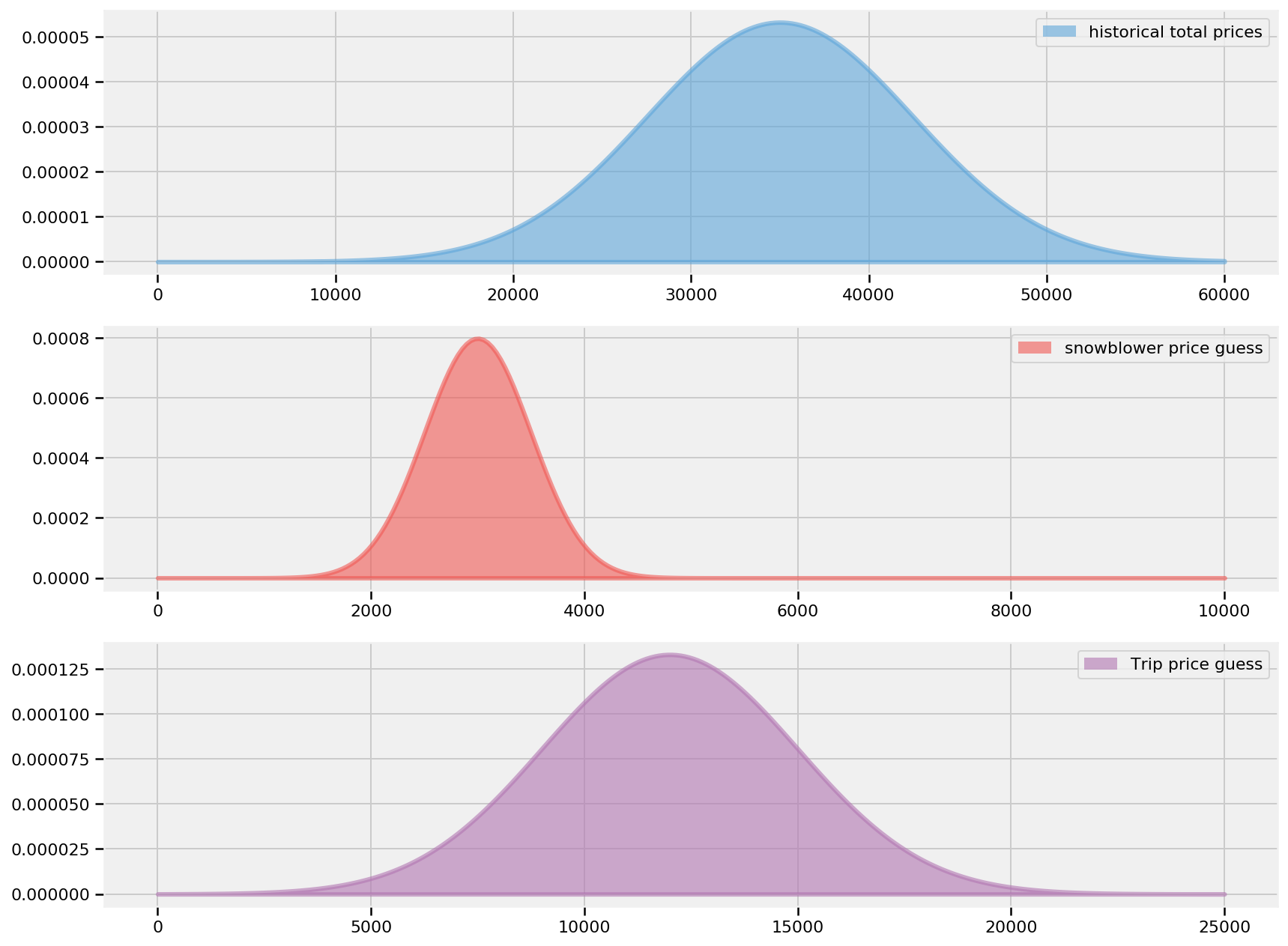In : data_mu = [3000., 12000.] data_std = [500., 3000.] mu_prior = 35000. std_prior = 7500. def posterior_log_prob(true_price, prize_1, prize_2): """ Our posterior log probability, as a function of states Args: true_price_: scalar of true price estimate, taken from state prize_1_: scalar of prize 1 estimate, to be added to the prize 1 estimate, taken from state prize_2_: scalar of prize 2 estimate, to be added to the prize 1 estimate, taken from state Returns: Scalar sum of log probabilities Closure over: data_mu, data_std, mu_prior, std_prior """ rv_true_price = tfd.Normal(loc=mu_prior, scale=std_prior, name="true_price") rv_prize_1 = tfd.Normal(loc=data_mu, scale=data_std, name="first_prize") rv_prize_2 = tfd.Normal(loc=data_mu, scale=data_std, name="second_prize") price_estimate = prize_1 + prize_2 rv_error = tfd.Normal(loc=price_estimate, scale=3000., name='error') return ( rv_true_price.log_prob(true_price) + rv_prize_1.log_prob(prize_1) + rv_prize_2.log_prob(prize_2) + rv_error.log_prob(true_price) )  Nice. Now we'll evaluate the result with our evaluate() function and see if it matches our expectations. In : number_of_steps = 50000 burnin = 10000 [ true_price, prize_1, prize_2 ], kernel_results = tfp.mcmc.sample_chain( num_results=number_of_steps, num_burnin_steps=burnin, current_state=[ tf.fill(, 20000., name='init_true_price'), tf.fill(, 3000., name='init_prize_1'), tf.fill(, 12000., name='init_prize_2') ], kernel=tfp.mcmc.RandomWalkMetropolis( new_state_fn=tfp.mcmc.random_walk_normal_fn(1000.), #specify a new callable that has the appropriate step size target_log_prob_fn=posterior_log_prob, seed=54), parallel_iterations=1, name='MCMC_eval') posterior_price_predictive_samples = true_price[:,0]  WARNING:tensorflow:From /usr/local/lib/python3.6/dist-packages/tensorflow_probability/python/mcmc/internal/util.py:164: where (from tensorflow.python.ops.array_ops) is deprecated and will be removed in a future version. Instructions for updating: Use tf.where in 2.0, which has the same broadcast rule as np.where  In : # performing our computations # Can take up to 2 minutes in Graph Mode [ posterior_price_predictive_samples_, kernel_results_, ] = evaluate([ posterior_price_predictive_samples, kernel_results, ]) # For metropolis hastings the acceptance probability should be around 0.234. # See https://arxiv.org/pdf/1011.6217.pdf print("acceptance rate: {}".format( kernel_results_.is_accepted.mean())) print("posterior_price_predictive_sample_ trace:", posterior_price_predictive_samples_)  acceptance rate: 0.44302 posterior_price_predictive_sample_ trace: [15410.804 15667.329 15667.329 ... 21354.152 21460.07 20892.367]  In : plt.figure(figsize(12.5, 4)) prices = tf.linspace(start=5000., stop=40000., num=35000) prior = tfd.Normal(loc=35000., scale=7500.).prob(prices) [ prices_, prior_, ] = evaluate([ prices, prior, ]) plt.plot(prices_, prior_, c="k", lw=2, label="prior dist. of suite price") hist = plt.hist(posterior_price_predictive_samples_, bins=35, normed=True, histtype="stepfilled") plt.title("Posterior of the true price estimate") plt.vlines(mu_prior, 0, 1.1 * np.max(hist), label="prior's mean", linestyles="--") plt.vlines(posterior_price_predictive_samples_.mean(), 0, 1.1 * np.max(hist), label="posterior's mean", linestyles="-.") plt.legend(loc="upper left");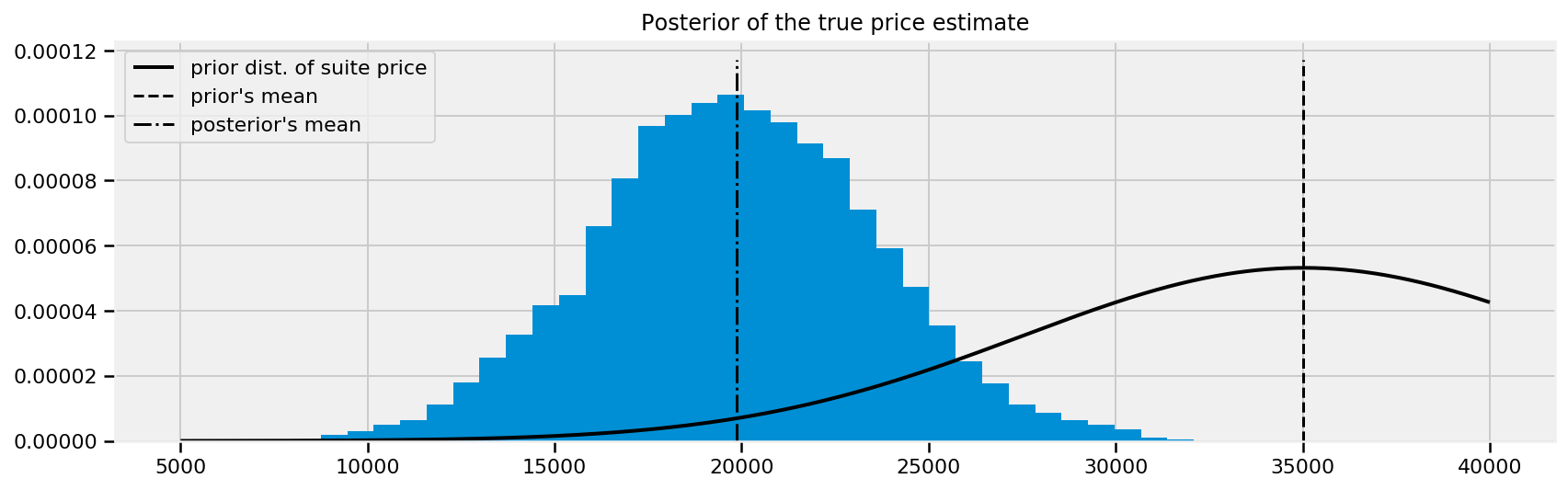Notice that because of our two observed prizes and subsequent guesses (including uncertainty about those guesses), we shifted our mean price estimate down about15 000 dollars from the previous mean price.

A frequentist, seeing the two prizes and having the same beliefs about their prices, would bid $\mu_1 + \mu_2 = 35000$, regardless of any uncertainty. Meanwhile, the naive Bayesian would simply pick the mean of the posterior distribution. But we have more information about our eventual outcomes; we should incorporate this into our bid. We will use the loss function above to find the best bid (best according to our loss).

What might a contestant's loss function look like? I would think it would look something like:

def showcase_loss(guess, true_price, risk=80000):
if true_price < guess:
return risk
elif abs(true_price - guess) <= 250:
return -2*np.abs(true_price)
else:
return np.abs(true_price - guess - 250)


where risk is a parameter that defines of how bad it is if your guess is over the true price. A lower risk means that you are more comfortable with the idea of going over. If we do bid under and the difference is less than $250, we receive both prizes (modeled here as receiving twice the original prize). Otherwise, when we bid under the true_price we want to be as close as possible, hence the else loss is a increasing function of the distance between the guess and true price. In : def showdown_loss(guess, size, true_price_, risk_ = 80000): """Stock Loss function. Args: guess: float32 Tensor, representing a range of guesses for the price, or one guess size: int size of guess true_price: float32 Tensor of size 50000 x num_guesses_, representing the prices from the HMC sampling, broadcast to each of the num_guesses_ guesses risk_: a scalar value representing a penalizer for a score going over (lower risk indicates more comfort with the price going over) Returns: loss: tensor of shape (true_price.shape,guess.shape), returning the loss function per definition in accompanying text """ true_price = tf.transpose(tf.broadcast_to(true_price_,(size,true_price_.shape))) risk = tf.broadcast_to (tf.convert_to_tensor(risk_,dtype=tf.float32),true_price.shape) return tf.where (true_price < guess , risk , \ tf.where(tf.abs(true_price - guess) <= 1,-2*tf.abs(true_price),tf.abs(true_price - guess -250))) num_guesses_ = 70 num_risks_ = 6 guesses = tf.linspace(5000., 50000., num_guesses_) risks_ = np.linspace(30000, 150000, num_risks_) results_cache_ = np.zeros ((num_risks_,num_guesses_)) expected_loss = lambda guess,size, risk: tf.reduce_mean( showdown_loss(guess,size, posterior_price_predictive_samples_, risk),axis=0) risk_num_ = 0 for _p in risks_: results = expected_loss(guesses,num_guesses_,tf.constant(_p,dtype=tf.float32)) [ guesses_ , results_ ] = evaluate([ guesses, results ]) plt.plot(guesses_, results_, label = "%d"%_p) results_cache_[risk_num_,:] = results_ risk_num_+=1 plt.title("Expected loss of different guesses, \nvarious risk-levels of \ overestimating") plt.legend(loc="upper left", title="Risk parameter") plt.xlabel("price bid") plt.ylabel("expected loss") plt.xlim(7000, 30000) plt.ylim(-1000, 80000);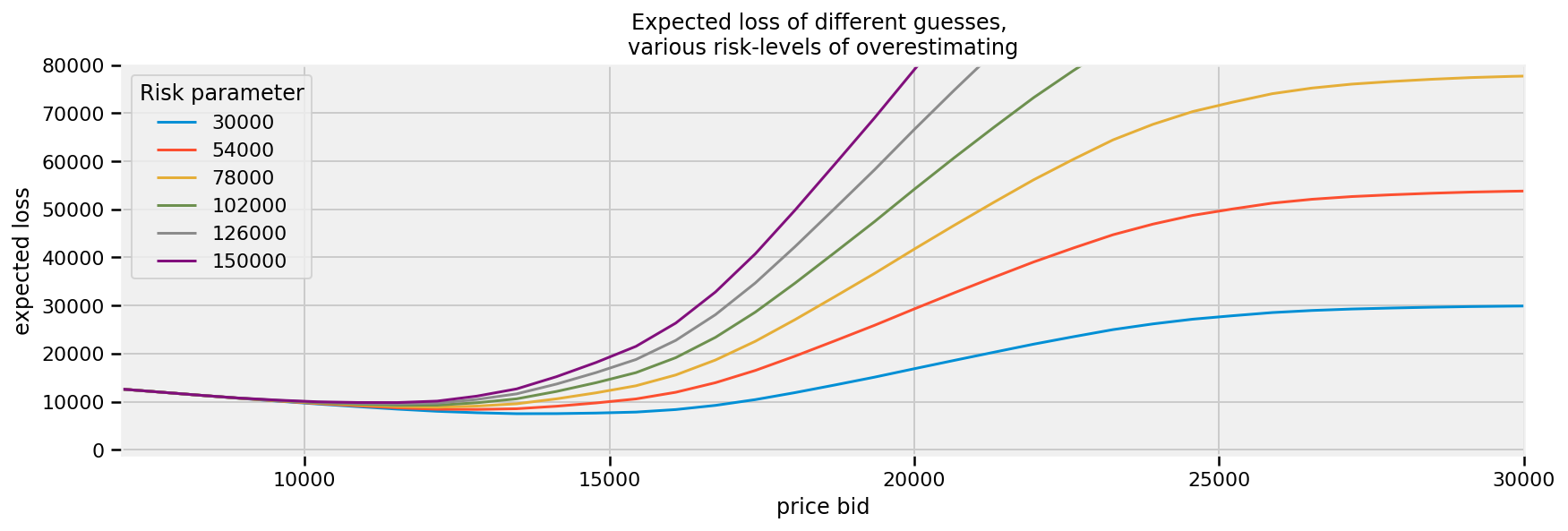For every possible bid, we calculate the expected loss associated with that bid. We vary the risk parameter to see how it affects our loss: ### Minimizing our losses¶ It would be wise to choose the estimate that minimizes our expected loss. This corresponds to the minimum point on each of the curves above. More formally, we would like to minimize our expected loss by finding the solution to $$\text{arg} \min_{\hat{\theta}} \;\;E_{\theta}\left[ \; L(\theta, \hat{\theta}) \; \right]$$ The minimum of the expected loss is called the Bayes action. We'll compute the minimum loss for the Showcase example above: In : ax = plt.subplot(111) risk_num_ = 0 for _p in risks_: color_ = next(ax._get_lines.prop_cycler) results_ = results_cache_[risk_num_,:] _g = tf.Variable(15000., trainable=True) loss = -expected_loss(_g,1, tf.constant(_p,dtype=tf.float32)) optimizer = tf.train.AdamOptimizer(10) opt_min = optimizer.minimize(loss, var_list=[_g]) evaluate(tf.global_variables_initializer()) min_losses = [] min_vals = [] for i in range(500): _, l, value_ = evaluate([opt_min, loss, _g]) min_losses.append(l) min_vals.append(value_) min_losses = np.asarray(min_losses) min_vals = np.asarray(min_vals) min_results_ = min_vals[np.argmax(min_losses)] plt.plot(guesses_, results_ , color = color_['color']) plt.scatter(min_results_, 0, s = 60, \ color= color_['color'], label = "%d"%_p) plt.vlines((min_results_), 0, 120000, color = color_['color'], linestyles="--") print("minimum at risk %d: %.2f" % (_p, (min_results_))) risk_num_ += 1 plt.title("Expected loss & Bayes actions of different guesses, \n \ various risk-levels of overestimating") plt.legend(loc="upper left", scatterpoints = 1, title = "Bayes action at risk:") plt.xlabel("price guess") plt.ylabel("expected loss") plt.xlim(7000, 30000) plt.ylim(-1000, 80000);  minimum at risk 30000: 13777.55 minimum at risk 54000: 12592.70 minimum at risk 78000: 12157.09 minimum at risk 102000: 12011.69 minimum at risk 126000: 11149.57 minimum at risk 150000: 11149.57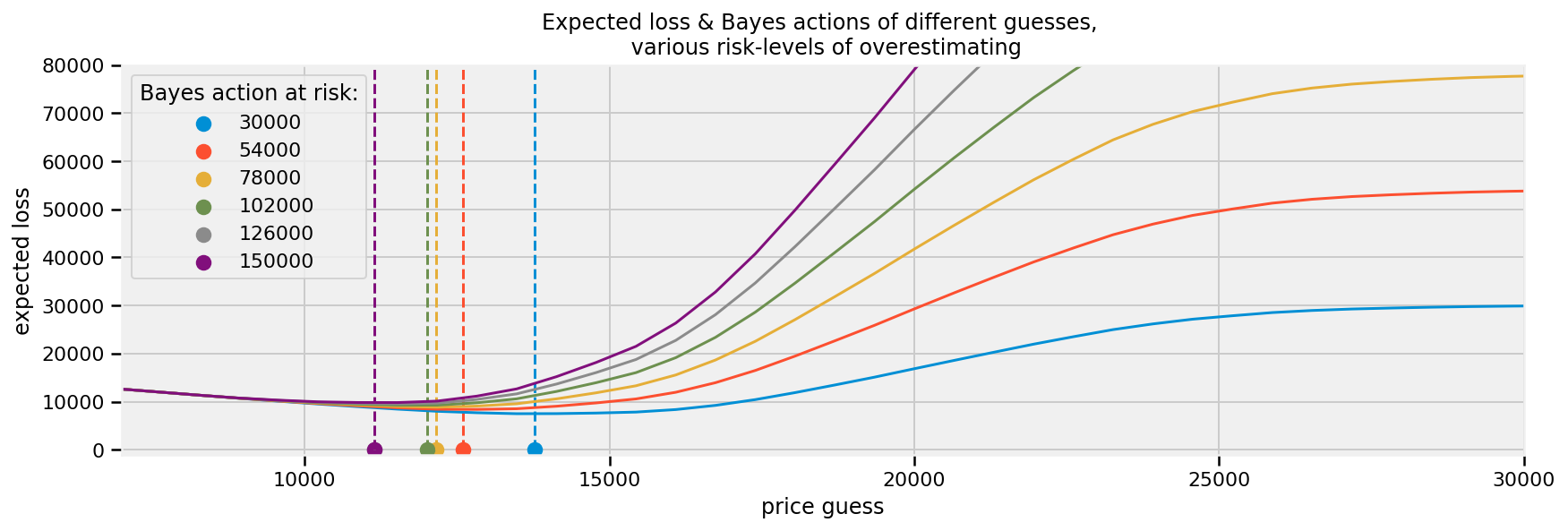As intuition suggests, as we decrease the risk threshold (care about overbidding less), we increase our bid, willing to edge closer to the true price. It is interesting how far away our optimized loss is from the posterior mean, which was about 20 000. Suffice to say, in higher dimensions being able to eyeball the minimum expected loss is impossible. ### Shortcuts¶ For some loss functions, the Bayes action is known in closed form. We list some of them below: • If using the mean-squared loss, the Bayes action is the mean of the posterior distribution, i.e. the value $$E_{\theta}\left[ \theta \right]$$ minimizes$E_{\theta}\left[ \; (\theta - \hat{\theta})^2 \; \right]$. Computationally this requires us to calculate the average of the posterior samples [See chapter 4 on The Law of Large Numbers] • Whereas the median of the posterior distribution minimizes the expected absolute-loss. The sample median of the posterior samples is an appropriate and very accurate approximation to the true median. • In fact, it is possible to show that the MAP estimate is the solution to using a loss function that shrinks to the zero-one loss. Maybe it is clear now why the first-introduced loss functions are used most often in the mathematics of Bayesian inference: no complicated optimizations are necessary. Luckily, we have machines to do the complications for us. ## Machine Learning via Bayesian Methods¶ Whereas frequentist methods strive to achieve the best precision about all possible parameters, machine learning cares to achieve the best prediction among all possible parameters. Of course, one way to achieve accurate predictions is to aim for accurate predictions, but often your prediction measure and what frequentist methods are optimizing for are very different. For example, least-squares linear regression is the most simple active machine learning algorithm. I say active as it engages in some learning, whereas predicting the sample mean is technically simpler, but is learning very little if anything. The loss that determines the coefficients of the regressors is a squared-error loss. On the other hand, if your prediction loss function (or score function, which is the negative loss) is not a squared-error, like AUC, ROC, precision, etc., your least-squares line will not be optimal for the prediction loss function. This can lead to prediction results that are suboptimal. Finding Bayes actions is equivalent to finding parameters that optimize not parameter accuracy but an arbitrary performance measure, however we wish to define performance (loss functions, AUC, ROC, precision/recall etc.). The next two examples demonstrate these ideas. The first example is a linear model where we can choose to predict using the least-squares loss or a novel, outcome-sensitive loss. The second example is adapted from a Kaggle data science project. The loss function associated with our predictions is incredibly complicated. # Example: Financial prediction¶ Suppose the future return of a stock price is very small, say 0.01 (or 1%). We have a model that predicts the stock's future price, and our profit and loss is directly tied to us acting on the prediction. How should we measure the loss associated with the model's predictions, and subsequent future predictions? A squared-error loss is agnostic to the signage and would penalize a prediction of -0.01 equally as bad a prediction of 0.03: $$(0.01 - (-0.01))^2 = (0.01 - 0.03)^2 = 0.004$$ If you had made a bet based on your model's prediction, you would have earned money with a prediction of 0.03, and lost money with a prediction of -0.01, yet our loss did not capture this. We need a better loss that takes into account the sign of the prediction and true value. We design a new loss that is better for financial applications below: In : plt.figure(figsize(12.5, 6.5)) reset_sess() def stock_loss(true_return, yhat, alpha=100.): """ Stock Loss function Args: true_return: float32 Tensor representing the true stock return yhat: float32 alpha:float32 Returns: float: absolute value of the difference between true_return and yhat """ if true_return * yhat < 0: # opposite signs, not good return alpha * yhat ** 2 - tf.sign(true_return) * yhat \ + tf.abs(true_return) else: return tf.abs(true_return - yhat) true_value_1_ = .05 true_value_2_ = -.02 pred_ = np.linspace(-.04, .12, 75) plt.plot(pred_, [evaluate(stock_loss(true_value_1_, p)) for p in pred_], label="Loss associated with\n prediction if true value = 0.05", lw=3) plt.vlines(0, 0, .25, linestyles="--") plt.xlabel("prediction") plt.ylabel("loss") plt.xlim(-0.04, .12) plt.ylim(0, 0.25) true_value = -.02 plt.plot(pred_, [evaluate(stock_loss(true_value_2_, p)) for p in pred_], alpha=0.6, label="Loss associated with\n prediction if true value = -0.02", lw=3) plt.legend() plt.title("Stock returns loss if true value = 0.05, -0.02");  Device mapping: /job:localhost/replica:0/task:0/device:XLA_CPU:0 -> device: XLA_CPU device /job:localhost/replica:0/task:0/device:XLA_GPU:0 -> device: XLA_GPU device /job:localhost/replica:0/task:0/device:GPU:0 -> device: 0, name: Tesla P100-PCIE-16GB, pci bus id: 0000:00:04.0, compute capability: 6.0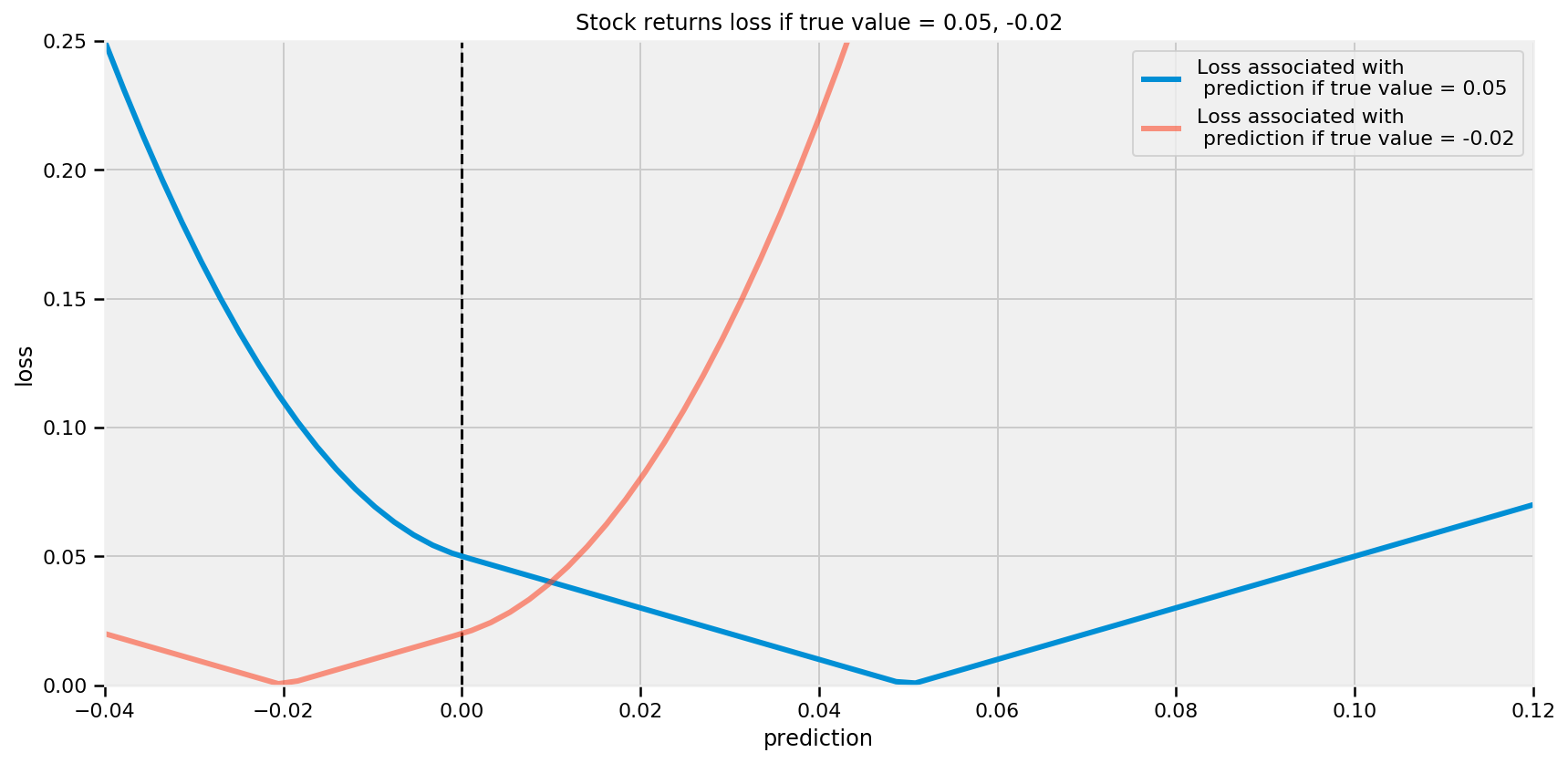Note the change in the shape of the loss as the prediction crosses zero. This loss reflects that the user really does not want to guess the wrong sign, especially be wrong and a large magnitude. Why would the user care about the magnitude? Why is the loss not 0 for predicting the correct sign? Surely, if the return is 0.01 and we bet millions we will still be (very) happy. Financial institutions treat downside risk, as in predicting a lot on the wrong side, and upside risk, as in predicting a lot on the right side, similarly. Both are seen as risky behaviour and discouraged. Hence why we have an increasing loss as we move further away from the true price. (With less extreme loss in the direction of the correct sign.) We will perform a regression on a trading signal that we believe predicts future returns well. Our dataset is artificial, as most financial data is not even close to linear. Below, we plot the data along with the least-squares line. In : # Code for creating artificial "dummy" data # This is a common strategy for testing our models # before applying it to real-world data reset_sess() # Resets the default tensorflow graph we're using num_data = 100 X_data = (0.025 * tfd.Normal(loc=0.,scale=1.).sample(sample_shape=num_data)) Y_data = (0.5 * X_data + 0.01 * tfd.Normal(loc=0.,scale=1.).sample(sample_shape=num_data)) tf_var_data = tf.nn.moments(X_data, axes=0) covar = tfp.stats.covariance(X_data,Y_data, sample_axis=0, event_axis=None) ls_coef = covar / tf_var_data [ X_data_, Y_data_, ls_coef_, ] = evaluate([ X_data, Y_data, ls_coef, ]) ls_intercept_ = Y_data_.mean() - ls_coef_ * X_data_.mean() plt.figure(figsize(12.5, 7)) plt.scatter(X_data_, Y_data_, c="k") plt.xlabel("trading signal") plt.ylabel("returns") plt.title("Empirical returns vs trading signal") plt.plot(X_data_, ls_coef_ * X_data_ + ls_intercept_, label="Least-squares line") plt.xlim(X_data_.min(), X_data_.max()) plt.ylim(Y_data_.min(), Y_data_.max()) plt.legend(loc="upper left");  Device mapping: /job:localhost/replica:0/task:0/device:XLA_CPU:0 -> device: XLA_CPU device /job:localhost/replica:0/task:0/device:XLA_GPU:0 -> device: XLA_GPU device /job:localhost/replica:0/task:0/device:GPU:0 -> device: 0, name: Tesla P100-PCIE-16GB, pci bus id: 0000:00:04.0, compute capability: 6.0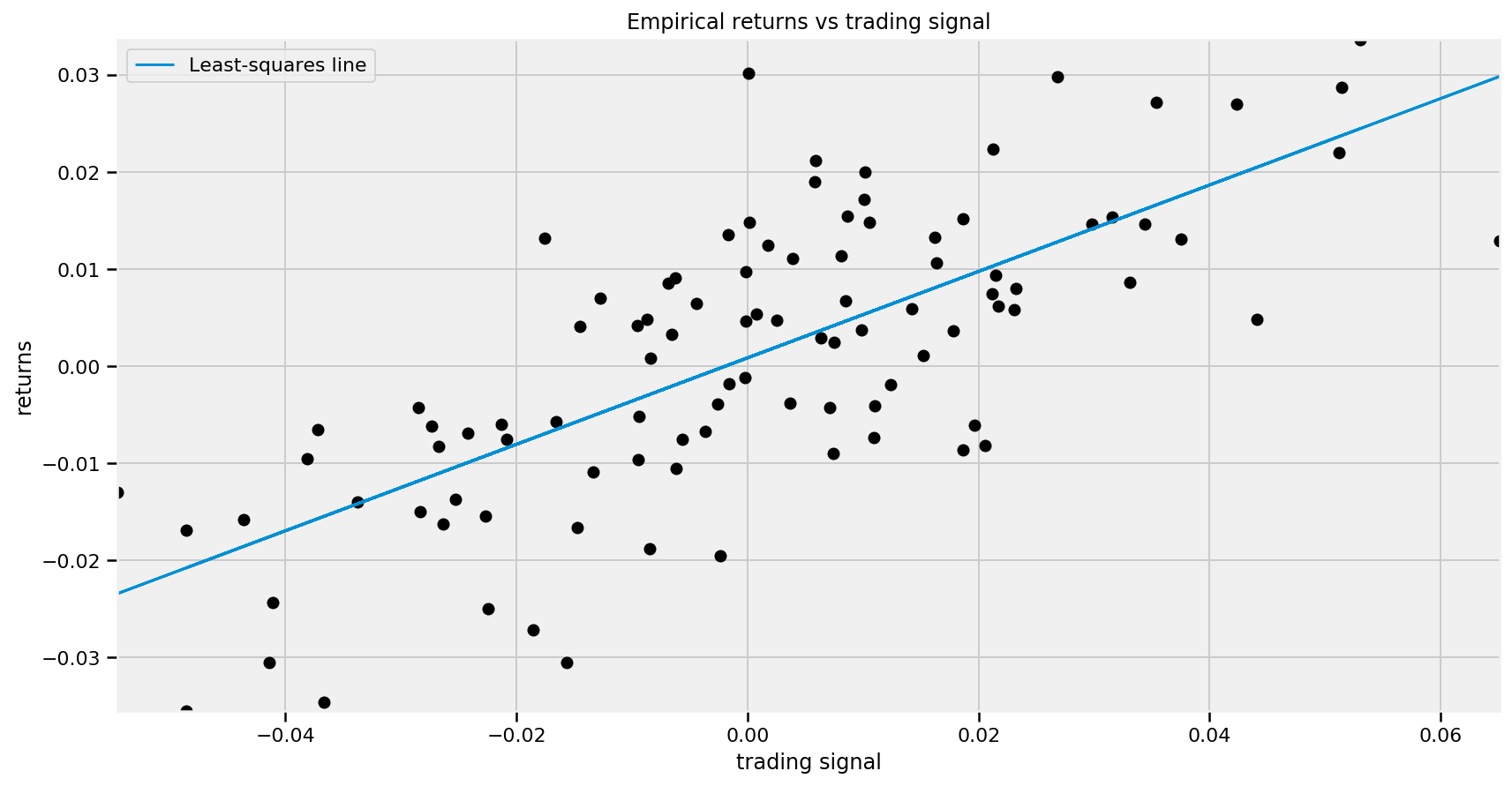We perform a simple Bayesian linear regression on this dataset. We look for a model like: $$R = \alpha + \beta x + \epsilon$$ where$\alpha, \beta$are our unknown parameters and$\epsilon \sim \text{Normal}(0, 1/\tau)$. The most common priors on$\beta$and$\alpha$are Normal priors. We will also assign a prior on$\tau$, so that$\sigma = 1/\sqrt{\tau}$is uniform over 0 to 100 (equivalently then$\tau = 1/\text{Uniform}(0, 100)^2\$).

In :
obs_stdev = tf.sqrt(
tf.reduce_mean(tf.squared_difference(Y_data_, tf.reduce_mean(Y_data_, axis=0)),
axis=0))

# Let's define the log probability of the bayesian regression function
def finance_posterior_log_prob(X_data_, Y_data_, alpha, beta, sigma):
"""
Our posterior log probability, as a function of states

Args:
alpha_: scalar, taken from state of the HMC
beta_: scalar, taken from state of the HMC
sigma_: scalar, the standard deviation of , taken from state of the HMC
Returns:
Scalar sum of log probabilities
Closure over: Y_data, X_data
"""
rv_std = tfd.Uniform(name="std", low=0., high=100.)
rv_beta = tfd.Normal(name="beta", loc=0., scale=100.)
rv_alpha = tfd.Normal(name="alpha", loc=0., scale=100.)

mean = alpha + beta * X_data_
rv_observed = tfd.Normal(name="obs", loc=mean, scale=sigma)

return (
rv_alpha.log_prob(alpha)
+ rv_beta.log_prob(beta)
+ rv_std.log_prob(sigma)
+ tf.reduce_sum(rv_observed.log_prob(Y_data_))
)

In :
number_of_steps = 30000
burnin = 5000

# Set the chain's start state.
initial_chain_state = [
tf.cast(1.,dtype=tf.float32) * tf.ones([], name='init_alpha', dtype=tf.float32),
tf.cast(0.01,dtype=tf.float32) * tf.ones([], name='init_beta', dtype=tf.float32),
tf.cast(obs_stdev,dtype=tf.float32) * tf.ones([], name='init_sigma', dtype=tf.float32)
]

# Since HMC operates over unconstrained space, we need to transform the
# samples so they live in real-space.
# Beta and sigma are 100x and 10x of alpha, approximately, so apply Affine scalar bijector
# to multiply the unconstrained beta and sigma by 100x and 10x to get back to
# the problem space
unconstraining_bijectors = [
tfp.bijectors.Identity(), #alpha
tfp.bijectors.AffineScalar(100.), #beta
tfp.bijectors.AffineScalar(10.),  #sigma
]

# Define a closure over our joint_log_prob.
unnormalized_posterior_log_prob = lambda *args: finance_posterior_log_prob(X_data_, Y_data_, *args)

# Initialize the step_size. (It will be automatically adapted.)
with tf.variable_scope(tf.get_variable_scope(), reuse=tf.AUTO_REUSE):
step_size = tf.get_variable(
name='step_size',
initializer=tf.constant(0.5, dtype=tf.float32),
trainable=False,
use_resource=True
)

# Defining the HMC
kernel=tfp.mcmc.TransformedTransitionKernel(
inner_kernel=tfp.mcmc.HamiltonianMonteCarlo(
target_log_prob_fn=unnormalized_posterior_log_prob,
num_leapfrog_steps=2,
step_size=step_size,
state_gradients_are_stopped=True),
bijector=unconstraining_bijectors)

kernel = tfp.mcmc.SimpleStepSizeAdaptation(
inner_kernel=kernel, num_adaptation_steps=int(burnin * 0.8))

# Sampling from the chain.
[
alpha,
beta,
sigma
], kernel_results = tfp.mcmc.sample_chain(
num_results = number_of_steps,
num_burnin_steps = burnin,
current_state=initial_chain_state,
kernel=kernel,
name='HMC_sampling'
)

# Initialize any created variables for preconditions
init_g = tf.global_variables_initializer()

WARNING:tensorflow:From /usr/local/lib/python3.6/dist-packages/tensorflow_core/python/ops/resource_variable_ops.py:1630: calling BaseResourceVariable.__init__ (from tensorflow.python.ops.resource_variable_ops) with constraint is deprecated and will be removed in a future version.
Instructions for updating:
If using Keras pass *_constraint arguments to layers.


Nice. Now we'll evaluate the result with our evaluate() function and see if it matches our expectations.

In :
# Running the Initializer on our model
evaluate(init_g)

# performing our computations
# can take up to about 4 mins in graph mode
[
alpha_,
beta_,
sigma_,
kernel_results_
] = evaluate([
alpha,
beta,
sigma,
kernel_results
])

print("acceptance rate: {}".format(
kernel_results_.inner_results.inner_results.is_accepted.mean()))

print("final step size: {}".format(
kernel_results_.new_step_size[-100:].mean()))

acceptance rate: 0.5614
final step size: 0.001251715118996799

In :
# plotting the Posterior Samples
plt.figure(figsize=(15,3))
plt.plot(np.arange(number_of_steps), sigma_, color=TFColor)
plt.title('HMC sigma (σ) convergence progression', fontsize=14)

plt.figure(figsize=(15,3))
plt.plot(np.arange(number_of_steps), beta_, color=TFColor)
plt.title('HMC beta (β) convergence progression', fontsize=14)

plt.figure(figsize=(15,3))
plt.plot(np.arange(number_of_steps), alpha_, color=TFColor)
plt.title('HMC alpha (α) convergence progression', fontsize=14)

Out:
Text(0.5, 1.0, 'HMC alpha (α) convergence progression')`